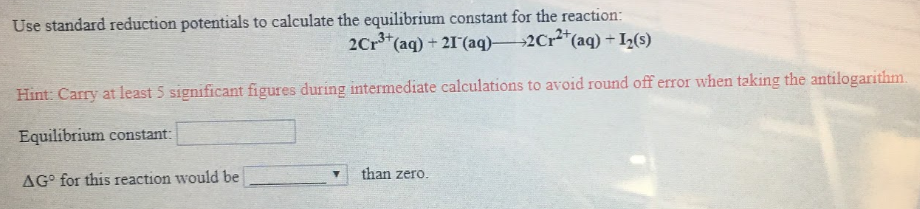# Use standard reduction potentials to calculate the equilibrium constant for the reaction: 2Cr^3+(aq) + 2I^-(aq) → 2Cr^2+(aq) + I2(s) Hint: Carry at least 5 significant figures during intermediate calculations to avoid round off error when taking the antilogarithm. Equilibrium constant: ΔG° for this reaction would be ____ than zero.